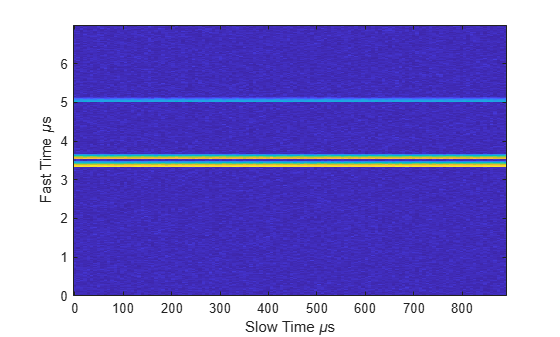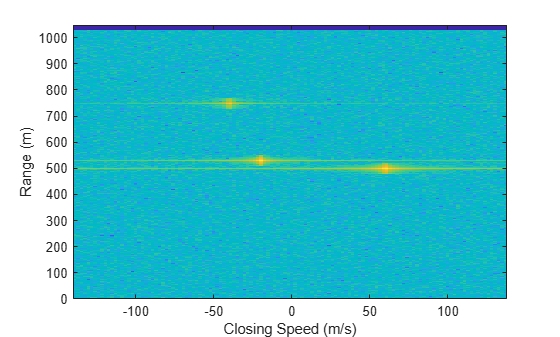# phased.DopplerEstimator

Doppler estimation

## Description

The phased.DopplerEstimator System object™ estimates Doppler frequencies of targets. Input to the estimator consists of detection locations output from a detector, and a range-Doppler response data cube. When detections are clustered, the Doppler frequencies are computed using cluster information. Clustering associates multiple detections into one extended detection.

To compute Doppler values for detections:

1. Define and set up your Doppler estimator using the Construction procedure that follows.

2. Call the step method to compute the Doppler of detections, using the properties you specify for the phased.DopplerEstimator System object.

Note

Instead of using the step method to perform the operation defined by the System object, you can call the object with arguments, as if it were a function. For example, y = step(obj,x) and y = obj(x) perform equivalent operations.

## Construction

estimator = phased.DopplerEstimator creates a Doppler estimator System object, estimator.

estimator = phased.DopplerEstimator(Name,Value) creates a System object, estimator, with each specified property Name set to the specified Value. You can specify additional name and value pair arguments in any order as (Name1,Value1,...,NameN,ValueN).

## Properties

expand all

Source of the number of requested Doppler estimates, specified as 'Auto' or 'Property'.

If you set this property to 'Auto', the number of estimates equals the number of columns in the detidx input argument of the step method. If cluster IDs are provided, the number of estimates equals the number of unique cluster IDs.

If you set this property to 'Property', the number of reported estimates is obtained from the value of the NumEstimates property.

Data Types: char

The maximum number of estimates to report, specified as a positive integer. When the number of requested estimates is greater than the number of columns in the detidx argument of the step method, the remainder is filled with NaN.

#### Dependencies

To enable this property, set the NumEstimatesSource property to 'Property'.

Data Types: c | double

Option to accept clusterids as an input argument to the step method, specified as false or true. Setting this property to true enables the clusterid input argument of the step method.

Data Types: logical

Option to enable output of Doppler variance estimate, specified as false or true. Doppler variances estimates are returned in the dopvar output argument of the step method.

Data Types: logical

The number of pulses in the Doppler processed data cube, specified as a positive integer.

#### Dependencies

To enable this property, set the VarianceOutputPort property to true.

Data Types: single | double

Source of noise power values, specified as 'Property' or 'Input port'. Noise power is used to compute Doppler estimation variance and SNR. If you set this property to 'Property', the value of the NoisePower property represents the noise power at the detection locations. If you set this property to 'Input port', you can specify noise power using the noisepower input argument of the step method.

Data Types: char

Constant noise power value over the range-Doppler data cube, specified as a positive scalar. Noise power units are linear. The same noise power value is applied to all detections.

#### Dependencies

To enable this property, set the VarianceOutputPort property to true and set NoisePowerSource to 'Property'.

Data Types: single | double

## Methods

 step Estimate target Doppler
Common to All System Objects
release

Allow System object property value changes

## Examples

collapse all

To estimate the range and speed of three targets, create a range-Doppler map using the phased.RangeDopplerResponse System object™. Then use the phased.RangeEstimator and phased.DopplerEstimator System objects to estimate range and speed. The transmitter and receiver are collocated isotropic antenna elements forming a monostatic radar system.

The transmitted signal is a linear FM waveform with a pulse repetition interval (PRI) of 7.0 μs and a duty cycle of 2%. The operating frequency is 77 GHz and the sample rate is 150 MHz.

fs = 150e6; c = physconst('LightSpeed'); fc = 77.0e9; pri = 7e-6; prf = 1/pri;

Set up the scenario parameters. The transmitter and receiver are stationary and located at the origin. The targets are 500, 530, and 750 meters from the radar along the x-axis. The targets move along the x-axis at speeds of –60, 20, and 40 m/s. All three targets have a nonfluctuating radar cross-section (RCS) of 10 dB. Create the target and radar platforms.

Numtgts = 3; tgtpos = zeros(Numtgts); tgtpos(1,:) = [500 530 750]; tgtvel = zeros(3,Numtgts); tgtvel(1,:) = [-60 20 40]; tgtrcs = db2pow(10)*[1 1 1]; tgtmotion = phased.Platform(tgtpos,tgtvel); target = phased.RadarTarget('PropagationSpeed',c,'OperatingFrequency',fc, ... 'MeanRCS',tgtrcs); radarpos = [0;0;0]; radarvel = [0;0;0]; radarmotion = phased.Platform(radarpos,radarvel);

Create the transmitter and receiver antennas.

txantenna = phased.IsotropicAntennaElement; rxantenna = clone(txantenna);

Set up the transmitter-end signal processing. Create an upsweep linear FM signal with a bandwidth of one half the sample rate. Find the length of the PRI in samples and then estimate the rms bandwidth and range resolution.

bw = fs/2; waveform = phased.LinearFMWaveform('SampleRate',fs, ... 'PRF',prf,'OutputFormat','Pulses','NumPulses',1,'SweepBandwidth',fs/2, ... 'DurationSpecification','Duty cycle','DutyCycle',0.02); sig = waveform(); Nr = length(sig); bwrms = bandwidth(waveform)/sqrt(12); rngrms = c/bwrms;

Set up the transmitter and radiator System object properties. The peak output power is 10 W and the transmitter gain is 36 dB.

peakpower = 10; txgain = 36.0; txgain = 36.0; transmitter = phased.Transmitter( ... 'PeakPower',peakpower, ... 'Gain',txgain, ... 'InUseOutputPort',true); radiator = phased.Radiator( ... 'Sensor',txantenna,... 'PropagationSpeed',c,... 'OperatingFrequency',fc);

Set up the free-space channel in two-way propagation mode.

channel = phased.FreeSpace( ... 'SampleRate',fs, ... 'PropagationSpeed',c, ... 'OperatingFrequency',fc, ... 'TwoWayPropagation',true);

Set up the receiver-end processing. Set the receiver gain and noise figure.

collector = phased.Collector( ... 'Sensor',rxantenna, ... 'PropagationSpeed',c, ... 'OperatingFrequency',fc); rxgain = 42.0; noisefig = 1; receiver = phased.ReceiverPreamp( ... 'SampleRate',fs, ... 'Gain',rxgain, ... 'NoiseFigure',noisefig);

Loop over the pulses to create a data cube of 128 pulses. For each step of the loop, move the target and propagate the signal. Then put the received signal into the data cube. The data cube contains the received signal per pulse. Ordinarily, a data cube has three dimensions where the last dimension corresponds to antennas or beams. Because only one sensor is used, the cube has only two dimensions.

The processing steps are:

1. Move the radar and targets.

2. Transmit a waveform.

3. Propagate the waveform signal to the target.

4. Reflect the signal from the target.

5. Propagate the waveform back to the radar. Two-way propagation enables you to combine the return propagation with the outbound propagation.

7. Load the signal into the data cube.

Np = 128; dt = pri; cube = zeros(Nr,Np); for n = 1:Np [sensorpos,sensorvel] = radarmotion(dt); [tgtpos,tgtvel] = tgtmotion(dt); [tgtrng,tgtang] = rangeangle(tgtpos,sensorpos); sig = waveform(); [txsig,txstatus] = transmitter(sig); txsig = radiator(txsig,tgtang); txsig = channel(txsig,sensorpos,tgtpos,sensorvel,tgtvel); tgtsig = target(txsig); rxcol = collector(tgtsig,tgtang); rxsig = receiver(rxcol); cube(:,n) = rxsig; end

Display the data cube containing signals per pulse.

imagesc([0:(Np-1)]*pri*1e6,[0:(Nr-1)]/fs*1e6,abs(cube)) xlabel('Slow Time {\mu}s') ylabel('Fast Time {\mu}s') axis xyCreate and display the range-Doppler image for 128 Doppler bins. The image shows range vertically and speed horizontally. Use the linear FM waveform for match filtering. The image is here is the range-Doppler map.

ndop = 128; rangedopresp = phased.RangeDopplerResponse('SampleRate',fs, ... 'PropagationSpeed',c,'DopplerFFTLengthSource','Property', ... 'DopplerFFTLength',ndop,'DopplerOutput','Speed', ... 'OperatingFrequency',fc); matchingcoeff = getMatchedFilter(waveform); [rngdopresp,rnggrid,dopgrid] = rangedopresp(cube,matchingcoeff); imagesc(dopgrid,rnggrid,10*log10(abs(rngdopresp))) xlabel('Closing Speed (m/s)') ylabel('Range (m)') axis xyBecause the targets lie along the positive x-axis, positive velocity in the global coordinate system corresponds to negative closing speed. Negative velocity in the global coordinate system corresponds to positive closing speed.

Estimate the noise power after matched filtering. Create a constant noise background image for simulation purposes.

mfgain = matchingcoeff'*matchingcoeff; dopgain = Np; noisebw = fs; noisepower = noisepow(noisebw,receiver.NoiseFigure,receiver.ReferenceTemperature); noisepowerprc = mfgain*dopgain*noisepower; noise = noisepowerprc*ones(size(rngdopresp));

Create the range and Doppler estimator objects.

rangeestimator = phased.RangeEstimator('NumEstimatesSource','Auto', ... 'VarianceOutputPort',true,'NoisePowerSource','Input port', ... 'RMSResolution',rngrms); dopestimator = phased.DopplerEstimator('VarianceOutputPort',true, ... 'NoisePowerSource','Input port','NumPulses',Np);

Locate the target indices in the range-Doppler image. Instead of using a CFAR detector, for simplicity, use the known locations and speeds of the targets to obtain the corresponding index in the range-Doppler image.

detidx = NaN(2,Numtgts); tgtrng = rangeangle(tgtpos,radarpos); tgtspd = radialspeed(tgtpos,tgtvel,radarpos,radarvel); tgtdop = 2*speed2dop(tgtspd,c/fc); for m = 1:numel(tgtrng) [~,iMin] = min(abs(rnggrid-tgtrng(m))); detidx(1,m) = iMin; [~,iMin] = min(abs(dopgrid-tgtspd(m))); detidx(2,m) = iMin; end

Find the noise power at the detection locations.

ind = sub2ind(size(noise),detidx(1,:),detidx(2,:));

Estimate the range and range variance at the detection locations. The estimated ranges agree with the postulated ranges.

[rngest,rngvar] = rangeestimator(rngdopresp,rnggrid,detidx,noise(ind))
rngest = 3×1 499.7911 529.8380 750.0983 
rngvar = 3×1 10-4 × 0.0273 0.0276 0.2094 

Estimate the speed and speed variance at the detection locations. The estimated speeds agree with the predicted speeds.

[spdest,spdvar] = dopestimator(rngdopresp,dopgrid,detidx,noise(ind))
spdest = 3×1 60.5241 -19.6167 -39.5838 
spdvar = 3×1 10-5 × 0.0806 0.0816 0.6188 

expand all

## References

 Richards, M. Fundamentals of Radar Signal Processing. 2nd ed. McGraw-Hill Professional Engineering, 2014.

 Richards, M., J. Scheer, and W. Holm, Principles of Modern Radar: Basic Principles. SciTech Publishing, 2010.

## Extended Capabilities

Introduced in R2017a